# C Program to implement Bucket sort Algorithm

In this tutorial you will learn about the C Program to implement Bucket sort Algorithm and its application with practical example.

## C Program to implement Bucket sort Algorithm

In this tutorial, we will learn to create a C program that will do Bucket sort using C programming.

## Prerequisites

Before starting with this tutorial we assume that you are best aware of the following C programming topics:

• Operators in C Programming.
• Basic Input and Output function in C Programming.
• Basic C programming.
• For loop in C Programming.
• Creating and Using the user-defined function in C programming.

## What is Bucket Sort?

In every programming language, the sorting of data is a very important factor.  In C Language. Many different techniques for sorting are available to work with but in this tutorial, we will focus on the BucketSort Technique.

In this technique, we will create multiple small groups containing a range of elements. Then these groups are back joint again and the sorted array is formed.

## Output:-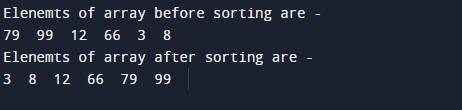In the above program, we have first declared and initialized a set of variables required in the program.

• n = it will hold the size of the array.
• x[]= it will hold the elements in an array.
• i = it will control the for loop for the array.

Function to print array:-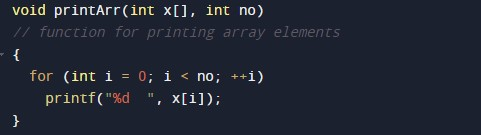Function for getting the max number.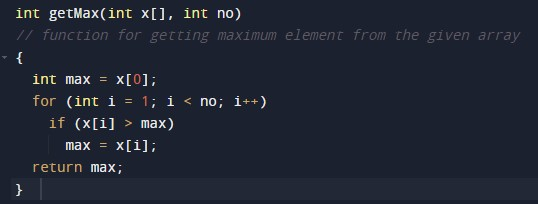Bucket sort function.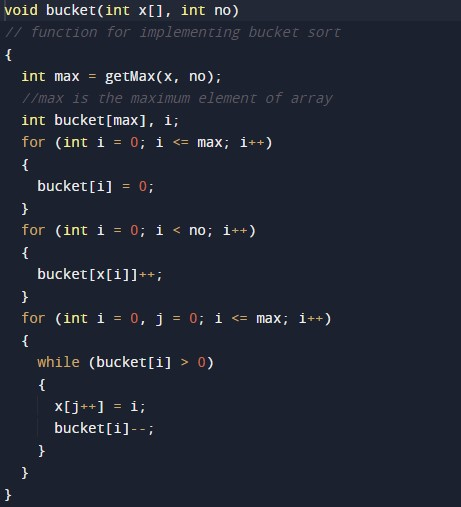Main function body.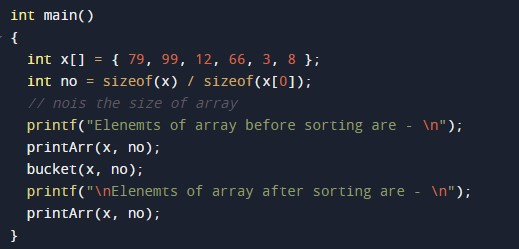In this tutorial we have learn about the C Program to implement Bucket sort Algorithm and its application with practical example. I hope you will like this tutorial.